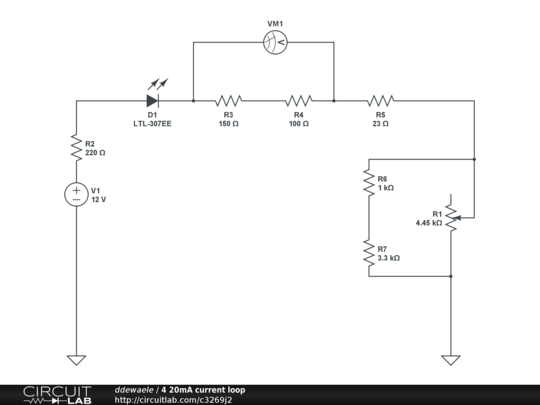# 4-20ma current loop simulator

I'm trying to create a simple 4-20mA current loop simulator that I can control using a potentiometer. The idea is to hook up this circuit to an Arduino and use the arduinos analog input to read in the current loop (by converting it into a 1-5V signal using a 250 ohm resistor). The potentiometer would be used to get a 4mA to 20mA current flowing through the circuit.

I've got a very simple circuit that is powered with 12V using an LED, some resistors and a potentiometer.

I figured I would get around 4mA when using a 2.7K resistor, and about 20mA when using a 560 ohm resistor.

I ended up with the following circuit. This kinda works, in the sense that If I turn the potentiometer to one side I get 4mA (1V) on one end , and 20mA (5V) on the other end, but it's not linear. the first 80% turn of the potentiometer gives me the 4mA - 10mA range, but then I only got a 20% turn left to getto 20mA.

How can I create a circuit that can get me from 4mA to 20mA in a linear way (using a potentiometer).• Are you using a LOG or LINEAR pot? – iggy Jan 3 '15 at 16:57
• Where is the wiper of the pot connected? As shown, the pot will have no effect. – Peter Bennett Jan 3 '15 at 17:06
• linear.... but using V=IxR it makes sense that R is not linear... V is always 12V-2V, Imin=0,004 and Imax=0,02. So to get from 0,004 to 0,005 you need 500 ohm difference. To get from 0,019 to 0,020 you need 26ohm. – ddewaele Jan 3 '15 at 17:09
• Error in schematic... Let me redraw.... Hope its correct now. I'm only using the middle and 1 outer pin. idea is when fully closed it's at 4,45K (with the 2 resistors in parallel this gives it about 2,3K giving me 4mA in the circuit). When fully open there's no resistance and I'm getting the full 20mA. – ddewaele Jan 3 '15 at 17:11

## 2 Answers

Are you using a LOG or LINEAR pot?

EDIT:

This is for the linear pot:Encircled in RED is your 10kOhm LINEAR potentiometer.

Resistance values may need a bit of tweaking.

EDIT2: this is an adjustable constant current source. Assuming the zener voltage is constant a constant current will flow through R1, R2, R3. The voltage on R1 will thus also be constant. This means the wiper of the potentiometer will travel between two fixed potentials. As the potentiometer is linear, the wiper voltage wil also change in a linear in fashion in relation to the actuation of the potentiometer. On the potentiometer wiper you have the base of Q1, which forms an emitor follower with R5. this prevents loading of the potentiometer. The voltage drop accross the base - emitor junction is approx. constant (~0.7V), which means the voltage accross R5 is also linear with the potentiometer travel. For practical purposes all the current flowing through R5 flows out the collector of Q1 and ends up in a load, the 250 Ohm resistor for your Arduino testing.

And there you go, your linear current with linear potentiometer travel.

• What's the theory behind this schematic ? (sorry still learning all of this stuff) – ddewaele Jan 3 '15 at 17:48
• Thx .. I don't have a zener diode at hand, only 1n4007 diodes. Would that work to ? I can get a 3,9 - 20,7 range if I use R1 = 2k2 and R5 = 300. But the resolution is a lot better when it is <10mA. As soon as it hts >10mA it becomes harder to get 0,1mA increments using the pot. – ddewaele Jan 4 '15 at 10:28
• No, a 1N4007 will not work. – iggy Jan 4 '15 at 13:44
• Just got the zener diode and the circuit works great ! Thanks a lot ... now to analyse it a bit. (might have a follow-up question for this in store). Thx. – ddewaele Jan 18 '15 at 11:09Try this. The extra diode helps to drop to 10V, and use a linear pot. If the voltage is still above 10V, use another diode, but most LEDs are 1.5 drop, it should work. When the pot is 0 Ohm, the current is 20mA. When the pot is 2k, the current is 4mA.

• but this will still give him 10mA at about 80% wiper setting... This is what he does not want. – iggy Jan 5 '15 at 15:55